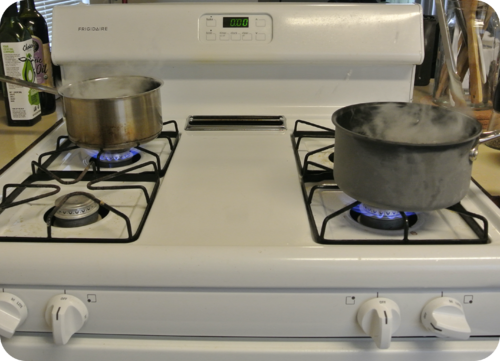# 11.13: Vapor Pressure Curves

$$\newcommand{\vecs}{\overset { \rightharpoonup} {\mathbf{#1}} }$$ $$\newcommand{\vecd}{\overset{-\!-\!\rightharpoonup}{\vphantom{a}\smash {#1}}}$$$$\newcommand{\id}{\mathrm{id}}$$ $$\newcommand{\Span}{\mathrm{span}}$$ $$\newcommand{\kernel}{\mathrm{null}\,}$$ $$\newcommand{\range}{\mathrm{range}\,}$$ $$\newcommand{\RealPart}{\mathrm{Re}}$$ $$\newcommand{\ImaginaryPart}{\mathrm{Im}}$$ $$\newcommand{\Argument}{\mathrm{Arg}}$$ $$\newcommand{\norm}{\| #1 \|}$$ $$\newcommand{\inner}{\langle #1, #2 \rangle}$$ $$\newcommand{\Span}{\mathrm{span}}$$ $$\newcommand{\id}{\mathrm{id}}$$ $$\newcommand{\Span}{\mathrm{span}}$$ $$\newcommand{\kernel}{\mathrm{null}\,}$$ $$\newcommand{\range}{\mathrm{range}\,}$$ $$\newcommand{\RealPart}{\mathrm{Re}}$$ $$\newcommand{\ImaginaryPart}{\mathrm{Im}}$$ $$\newcommand{\Argument}{\mathrm{Arg}}$$ $$\newcommand{\norm}{\| #1 \|}$$ $$\newcommand{\inner}{\langle #1, #2 \rangle}$$ $$\newcommand{\Span}{\mathrm{span}}$$$$\newcommand{\AA}{\unicode[.8,0]{x212B}}$$Figure $$\PageIndex{1}$$ (Credit: CK-12 Foundation; Source: CK-12 Foundation; License: CC BY-NC 3.0(opens in new window))

### What is the fastest way to boil water?

One of the first lessons in cooking is how to boil water. Yes, it sounds simple, but there are a couple of hints that speed things up. One hint is to put a lid on the pot. The picture above has water boiling uncovered with the steam escaping to the atmosphere. If the lid is on the pot, less water will be boiled off and the water will boil faster. The buildup of pressure inside the pot helps speed up the boiling process.

## Vapor Pressure Curves

The boiling points of various liquids can be illustrated in a vapor pressure curve (figure below). A vapor pressure curve is a graph of vapor pressure as a function of temperature. To find the normal boiling point of liquid, a horizontal line is drawn from the y-axis at a pressure equal to standard pressure. A vertical line starting at the x-axis can be drawn connected to the point at which the standard pressure and the vapor pressure curve of a liquid intersect—the corresponding temperature is the boiling point of that liquid.Figure $$\PageIndex{2}$$: Vapor pressure curves. (Credit: Christopher Auyeung; Source: CK-12 Foundation; License: CC BY-NC 3.0(opens in new window))

The boiling point of a liquid also correlates to the strength of its intermolecular forces. Recall that diethyl ether has weak dispersion forces, which means that the liquid has a high vapor pressure. The weak forces also mean that it does not require a large input of energy to make diethyl ether boil, and so it has a relatively low normal boiling point of $$34.6^\text{o} \text{C}$$. Water, with its much stronger hydrogen bonding, has a low vapor pressure and a higher normal boiling point of $$100^\text{o} \text{C}$$.

As stated earlier, boiling points are affected by external pressure. At higher altitudes, the atmospheric pressure is lower. With less pressure pushing down on the surface of the liquid, it boils at a lower temperature. This can also be seen from the vapor pressure curves. If one draws a horizontal line at a lower vapor pressure, it intersects each curve at a lower temperature. The boiling point of water is $$100^\text{o} \text{C}$$ at sea level, where the atmospheric pressure is standard. In Denver, Colorado at $$1600 \: \text{m}$$ above sea level, the atmospheric pressure is about $$640 \: \text{mm} \: \ce{Hg}$$ and water boils at about $$95^\text{o} \text{C}$$. On the summit of Mt. Everest, the atmospheric pressure is about $$255 \: \text{mm} \: \ce{Hg}$$, and water boils at only $$70^\text{o} \text{C}$$. On the other hand, water boils at greater than $$100^\text{o} \text{C}$$ if the external pressure is higher than standard. Pressure cookers do not allow the vapor to escape and the vapor pressure increases. Since water in a pressure cooker boils at a temperature above $$100^\text{o} \text{C}$$, the food cooks more quickly.Figure $$\PageIndex{3}$$: Pressure cooker. (Credit: Dinner Series; Source: Flickr, Dinner Series(opens in new window) [www.flickr.com]; License: CC by 2.0(opens in new window))

The effect of decreased air pressure can be demonstrated by placing a beaker of water in a vacuum chamber. At a low enough pressure, about $$20 \: \text{mm} \: \ce{Hg}$$, water will boil at room temperature.

## Summary

• A vapor pressure curve is a graph of vapor pressure as a function of temperature.
• Boiling points are affected by external pressure.

## Review

1. What does a vapor pressure curve show?
2. Why does diethyl ether have a low boiling point?
3. What intermolecular forces hold water molecules together?
4. Why does water boil at a lower temperature when at a high altitude?

## Explore More

Use the resource below to answer the questions that follow.

1. What does a vacuum pump do?
2. What is the role of the O-ring?
3. What happens when the vacuum pump is turned on?
4. What happened when the vacuum pump was turned on?
5. What was the temperature of this boiling water?

This page titled 11.13: Vapor Pressure Curves is shared under a CK-12 license and was authored, remixed, and/or curated by CK-12 Foundation via source content that was edited to the style and standards of the LibreTexts platform; a detailed edit history is available upon request.